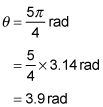##### Physics I: 501 Practice Problems For Dummies (+ Free Online Practice)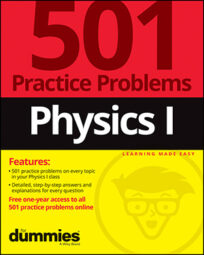In physics, it's useful to know how to calculate angles in radians. But first, you need to convert those angles from degrees to radians. The following practice questions walk you through the conversion process.

## Practice questions

1. You cut a cake into 8 equal pieces. What is the angle, in radians, of the pointed end of each piece of cake?

2. A drawbridge pivots upward from the horizontal position and moves through two-thirds of the angle between the horizontal and vertical positions. Through what angle (in radians) has the drawbridge moved?

3. Cleaning up after a party, you find leftover pizza slices. The pizzas were round, and the slices were all cut through the center. You find two slices that together make a quarter of a pizza and three slices that are each an eighth of a pizza. If you put all the slices side by side as in a pizza, what is the total angle made (in radians)?

The following are the answers to the practice questions:

A full circle has an angle of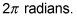To find the angle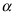that is one eighth of this, you must divide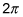by 8: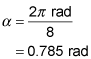2.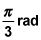The angle between horizontal and vertical is 90 degrees, or a quarter of a circle. Because there are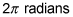in a circle, there are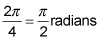in 90 degrees. The angle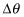through which the drawbridge moved is then two-thirds of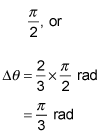You're told that the first two slices make a quarter of a whole pizza, which means that they make a quarter of a circle. There arein a circle, so this angle is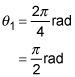The last three slices each make an eighth of a circle, so they each make an angle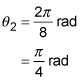The total angle made when the slices are laid side by side is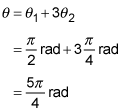Using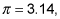this gives